# Instrumentation Classification of Instruments 1 Active Passive Instruments

• Slides: 17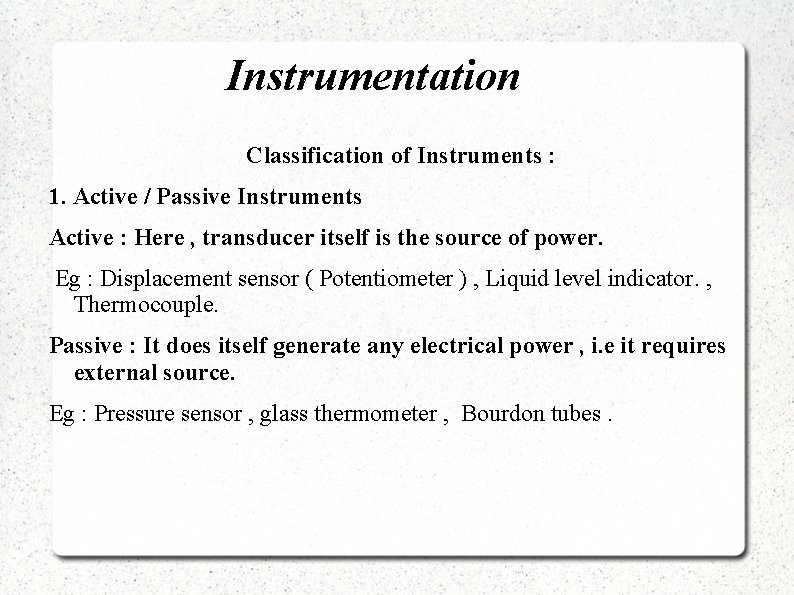Instrumentation Classification of Instruments : 1. Active / Passive Instruments Active : Here , transducer itself is the source of power. Eg : Displacement sensor ( Potentiometer ) , Liquid level indicator. , Thermocouple. Passive : It does itself generate any electrical power , i. e it requires external source. Eg : Pressure sensor , glass thermometer , Bourdon tubes.Instrumentation 2. Null / Deflection type : Null type : Here , a null / zero indication will lead to the determination of the magnitude of measured quantity. They have high accuracy and sensitivity. eg : dc potentiometer , Wheat stone's bridge. Deflection type : Here , deflection of the instrument forms basis of measurement. They are more suitable for measurements under dynamic conditions eg : PMMC ammeter , Wheat stone's bridge.Instrumentation 3. Monitoring / Transmitting Instruments : Monitoring type : eg : Flowmeter , LVDT , strain gauges. Transmitting type : eg : RTD , Thermocouple. Some Instruments can be both Monitoring and Transmitting type. 4. Analog / Digital Instruments :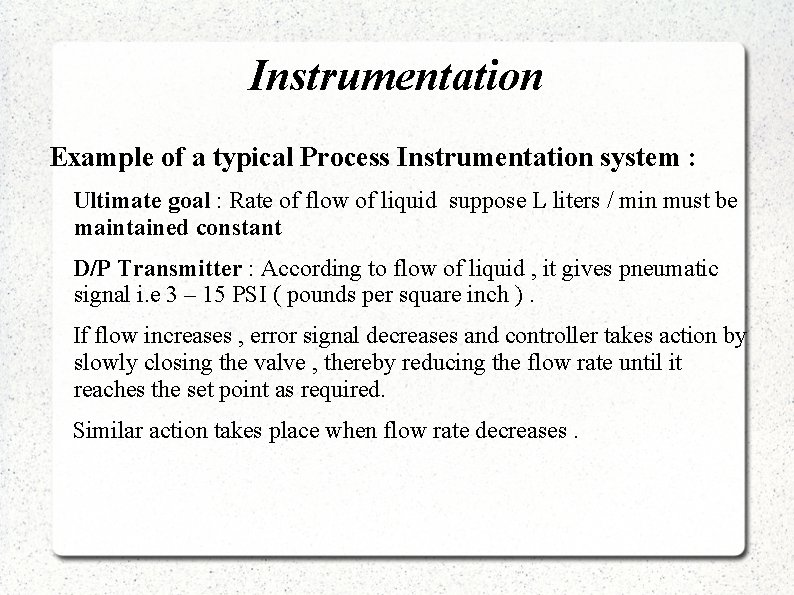Instrumentation Example of a typical Process Instrumentation system : Ultimate goal : Rate of flow of liquid suppose L liters / min must be maintained constant D/P Transmitter : According to flow of liquid , it gives pneumatic signal i. e 3 – 15 PSI ( pounds per square inch ). If flow increases , error signal decreases and controller takes action by slowly closing the valve , thereby reducing the flow rate until it reaches the set point as required. Similar action takes place when flow rate decreases.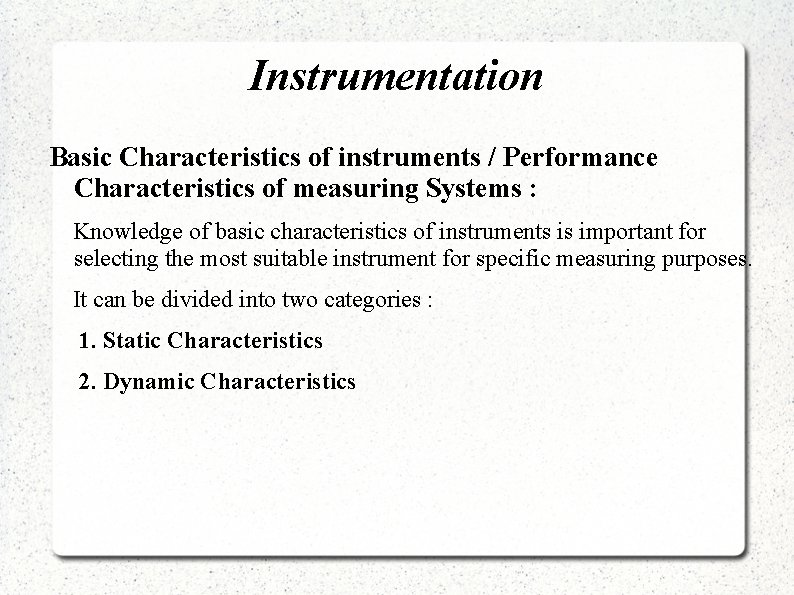Instrumentation Basic Characteristics of instruments / Performance Characteristics of measuring Systems : Knowledge of basic characteristics of instruments is important for selecting the most suitable instrument for specific measuring purposes. It can be divided into two categories : 1. Static Characteristics 2. Dynamic CharacteristicsInstrumentation Static Characteristics : Static Characteristics of an instrument are concerned only with steady state readings. Span If in a measuring instrument the highest point of calibration is x 2 units and the lowest point of calibration is x 1 units. Then the instrument range is x 2 units. The instrument span is given by : Span = ( x 2 – x 1 ) units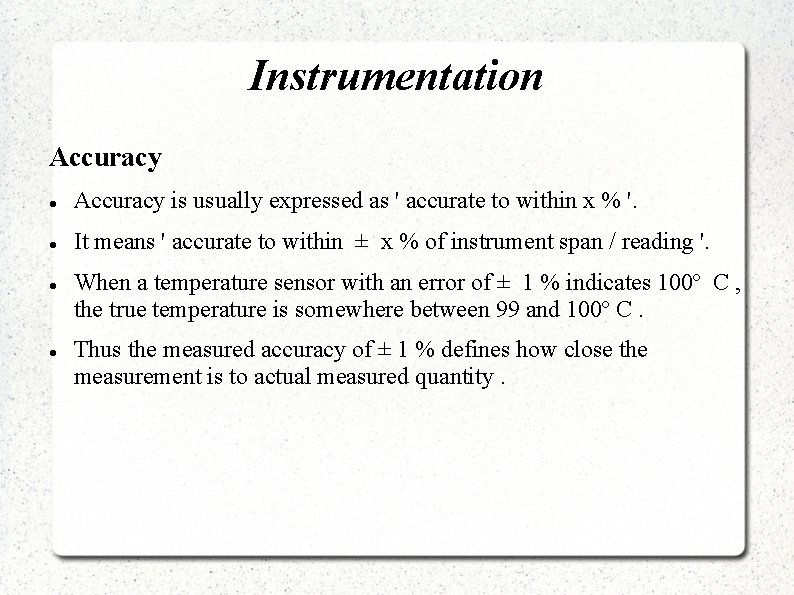Instrumentation Accuracy is usually expressed as ' accurate to within x % '. It means ' accurate to within ± x % of instrument span / reading '. When a temperature sensor with an error of ± 1 % indicates 100º C , the true temperature is somewhere between 99 and 100º C. Thus the measured accuracy of ± 1 % defines how close the measurement is to actual measured quantity.Instrumentation Precision is a measure of the degree to which successive measurements differ from each other. Linearity If the calibration curve of an instrument is not a straight line then it is not a linear instrument however it may be very accurate. It is normally desirable that the output reading of an instrument is linearly proportional to the quantity being measured. Non-linearity if defined as maximum deviation of any of the output readings marked with a crossed from this straight line and it is expressed as a % of full scale reading.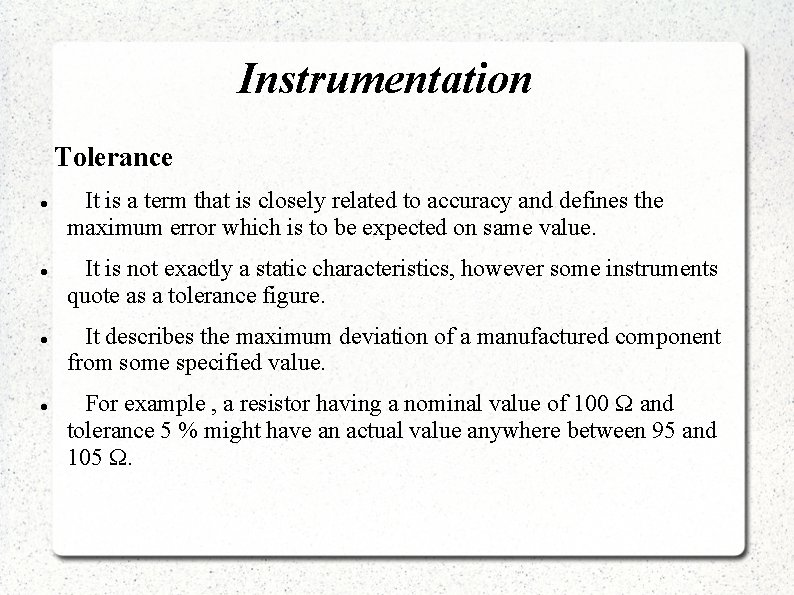Instrumentation Tolerance It is a term that is closely related to accuracy and defines the maximum error which is to be expected on same value. It is not exactly a static characteristics, however some instruments quote as a tolerance figure. It describes the maximum deviation of a manufactured component from some specified value. For example , a resistor having a nominal value of 100 Ω and tolerance 5 % might have an actual value anywhere between 95 and 105 Ω.Instrumentation Static Error It is the difference between the true value of a time invariant measurable quantity and the value indicated by the instrument. It is the deviation from the true value of the measured variable. For static error in units : True value + static error = Instrument reading or True value = Instrument reading – Static error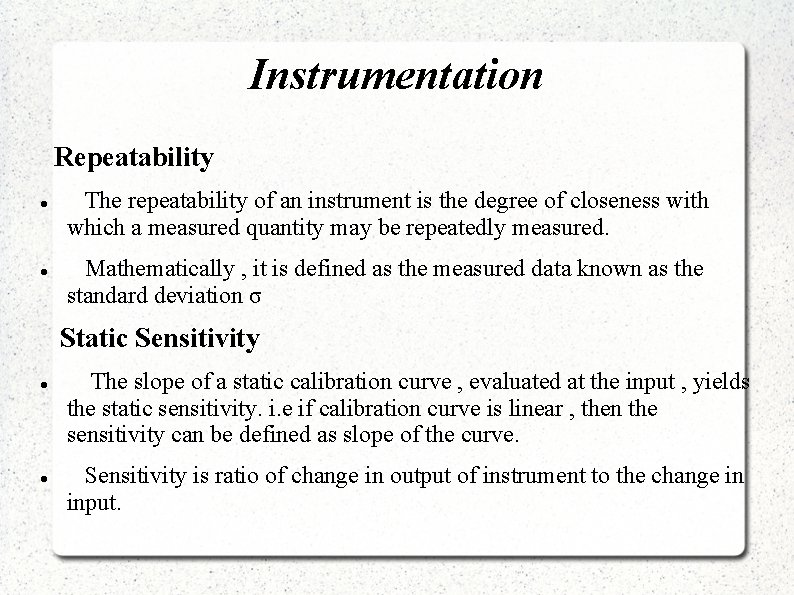Instrumentation Repeatability The repeatability of an instrument is the degree of closeness with which a measured quantity may be repeatedly measured. Mathematically , it is defined as the measured data known as the standard deviation σ Static Sensitivity The slope of a static calibration curve , evaluated at the input , yields the static sensitivity. i. e if calibration curve is linear , then the sensitivity can be defined as slope of the curve. Sensitivity is ratio of change in output of instrument to the change in input.Instrumentation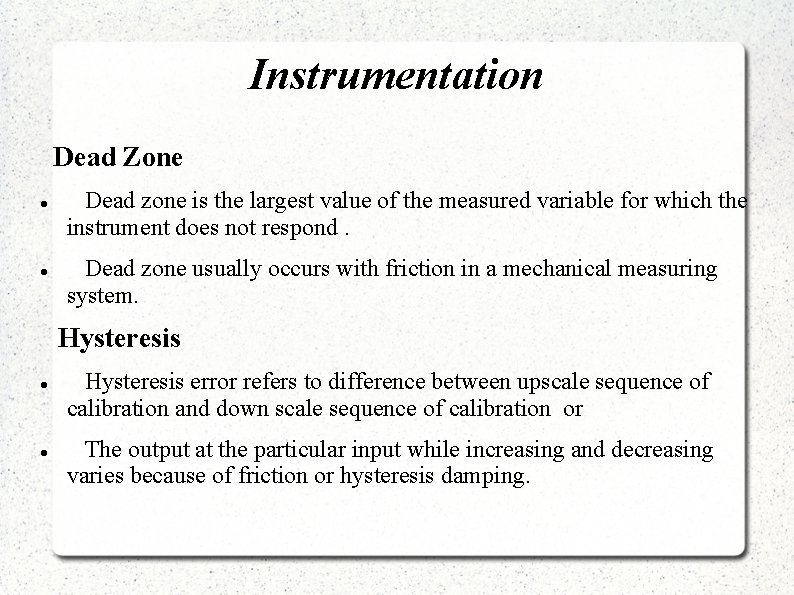Instrumentation Dead Zone Dead zone is the largest value of the measured variable for which the instrument does not respond. Dead zone usually occurs with friction in a mechanical measuring system. Hysteresis error refers to difference between upscale sequence of calibration and down scale sequence of calibration or The output at the particular input while increasing and decreasing varies because of friction or hysteresis damping.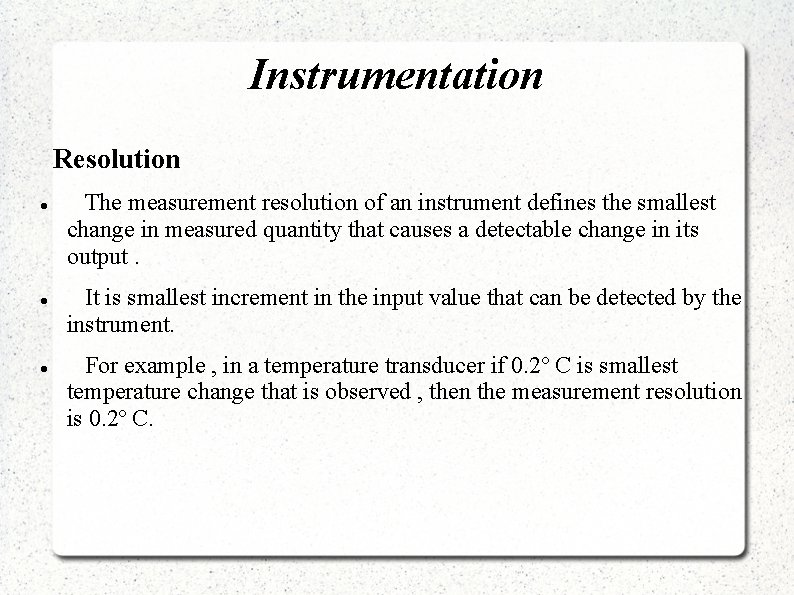Instrumentation Resolution The measurement resolution of an instrument defines the smallest change in measured quantity that causes a detectable change in its output. It is smallest increment in the input value that can be detected by the instrument. For example , in a temperature transducer if 0. 2º C is smallest temperature change that is observed , then the measurement resolution is 0. 2º C.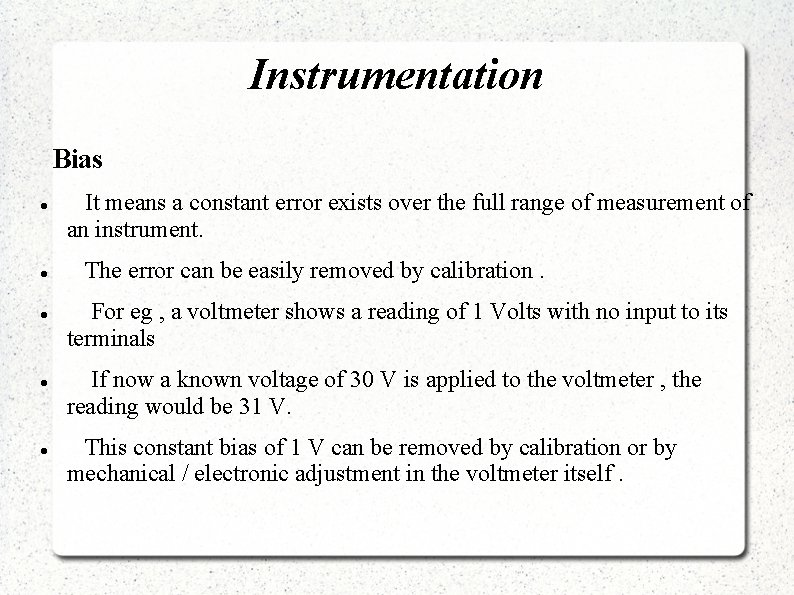Instrumentation Bias It means a constant error exists over the full range of measurement of an instrument. The error can be easily removed by calibration. For eg , a voltmeter shows a reading of 1 Volts with no input to its terminals If now a known voltage of 30 V is applied to the voltmeter , the reading would be 31 V. This constant bias of 1 V can be removed by calibration or by mechanical / electronic adjustment in the voltmeter itself.Instrumentation Drift The calibration of an instrument is usually performed under some controlled conditions of temperature , pressure , etc. As the variations occur in the ambient temperature etc , static characteristics change namely zero drift and sensitivity drift. Zero Drift It is the deviation of the output from its zero value , when the variable to be measured is constant. The effect of zero drift is to impose bias on the instrument. Sensitivity Drift It defines the amount by which an instrument sensitivity measurement varies as ambient conditions change.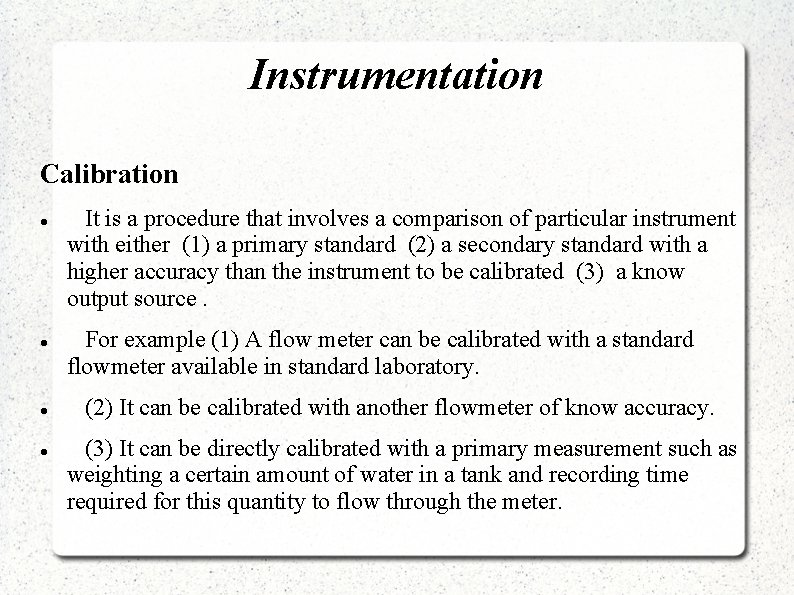Instrumentation Calibration It is a procedure that involves a comparison of particular instrument with either (1) a primary standard (2) a secondary standard with a higher accuracy than the instrument to be calibrated (3) a know output source. For example (1) A flow meter can be calibrated with a standard flowmeter available in standard laboratory. (2) It can be calibrated with another flowmeter of know accuracy. (3) It can be directly calibrated with a primary measurement such as weighting a certain amount of water in a tank and recording time required for this quantity to flow through the meter.